# How To Calculate and Solve for the Area, Radius and Diameter of a Circle | The Calculator Encyclopedia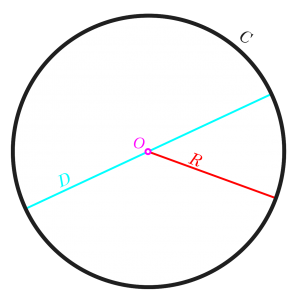The image above is a circle.

To compute the area of a circle, one essential parameter is needed and this parameter is the radius of a circle (r). You can also use the diameter of a circle to compute the area of a circle (d).

The formula for calculating the area of a circle is:

A = πr2

Where:

A = Area of a circle
r = Radius of a circle

Let’s solve an example:
Find the area of a circle where the radius of a circle is 6 cm.

This implies that;
r = Radius of a circle = 6 cm

A = πr2
A = 3.142 (6)2
A = 3.142 (36)
A = 113.10

Therefore, the area of a circle is 113.10 cm2

Calculating the Area of a Circle using the Diameter of a Circle.

The formula is A = πd2/4

Where;
A = Area of a circle
d = Diameter of a circle

Let’s solve an example:
Find the area of a circle where the diameter of the circle is 7 cm.

This implies that;
d = Diameter of a circle = 4 cm

A = πd2 / 4
A = 3.142 (4)2 / 4
A = 3.142 (16) / 4
A = 3.142 (4)
A = 12.57

Therefore, the area of a circle with diameter given is 12.57 cm2

How to Calculate Radius of a Circle when Area of the Circle is Given

r = √ (A / π )

where;

r = Radius of a circle
A = Area of a circle

Let’s solve an example:
Find the radius of a circle where the area of the circle is 16 cm2

This implies that;
A = Area of the circle = 16 cm2

r = √ (A / π)
r =√ (16 / π)
r = √ (16 / 3.142)
r = √ (5.09)
r  = 2.26

Therefore, the radius of the circle is 2.26 cm.

How to Calculate Diameter of a Circle when Area of the Circle is Given

d = √ (4A / π)

where;

d = Diameter of a circle
A = Area of a circle

Let’s solve an example;
Find the diameter of a circle where the area of the circle is 20 cm2

This implies that;
A = Area of the circle = 20 cm2

d = √ (4A / π)
d = √ (4(20) / π)
d = √ (80 / π)
d = √ (80 / 3.142)
d = √(25.46)
d = 5.046

Therefore, the diameter of the circle with area is 5.046 cm.

Nickzom Calculator – The Calculator Encyclopedia is capable of calculating the area of a circle.

To get the answer and workings of the area of a circle using the Nickzom Calculator – The Calculator Encyclopedia. First, you need to obtain the app.

You can get this app via any of these means:

To get access to the professional version via web, you need to register and subscribe for NGN 1,500 per annum to have utter access to all functionalities.
You can also try the demo version via https://www.nickzom.org/calculator

Once, you have obtained the calculator encyclopedia app, proceed to the Calculator Map, then click on Mensuration under the Mathematics section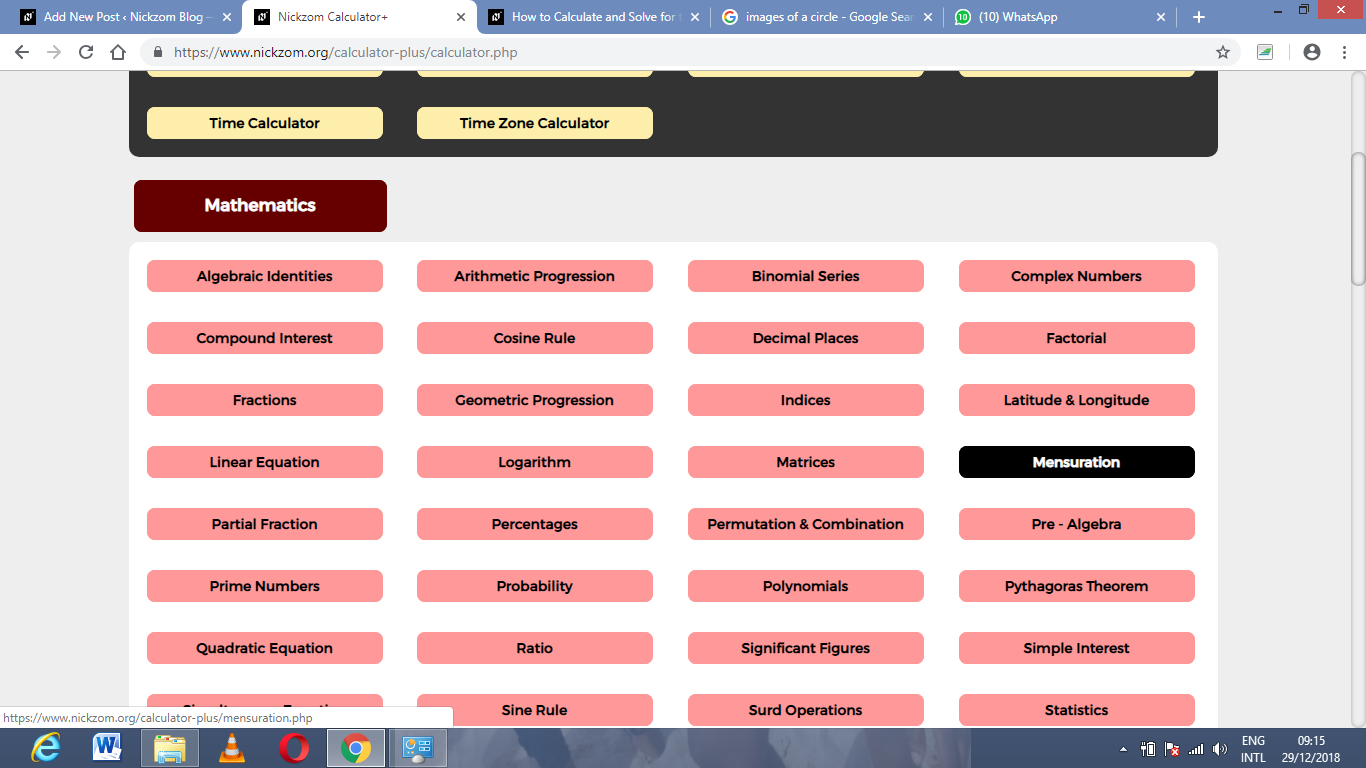Now, click on Area of a Circle under Mensuration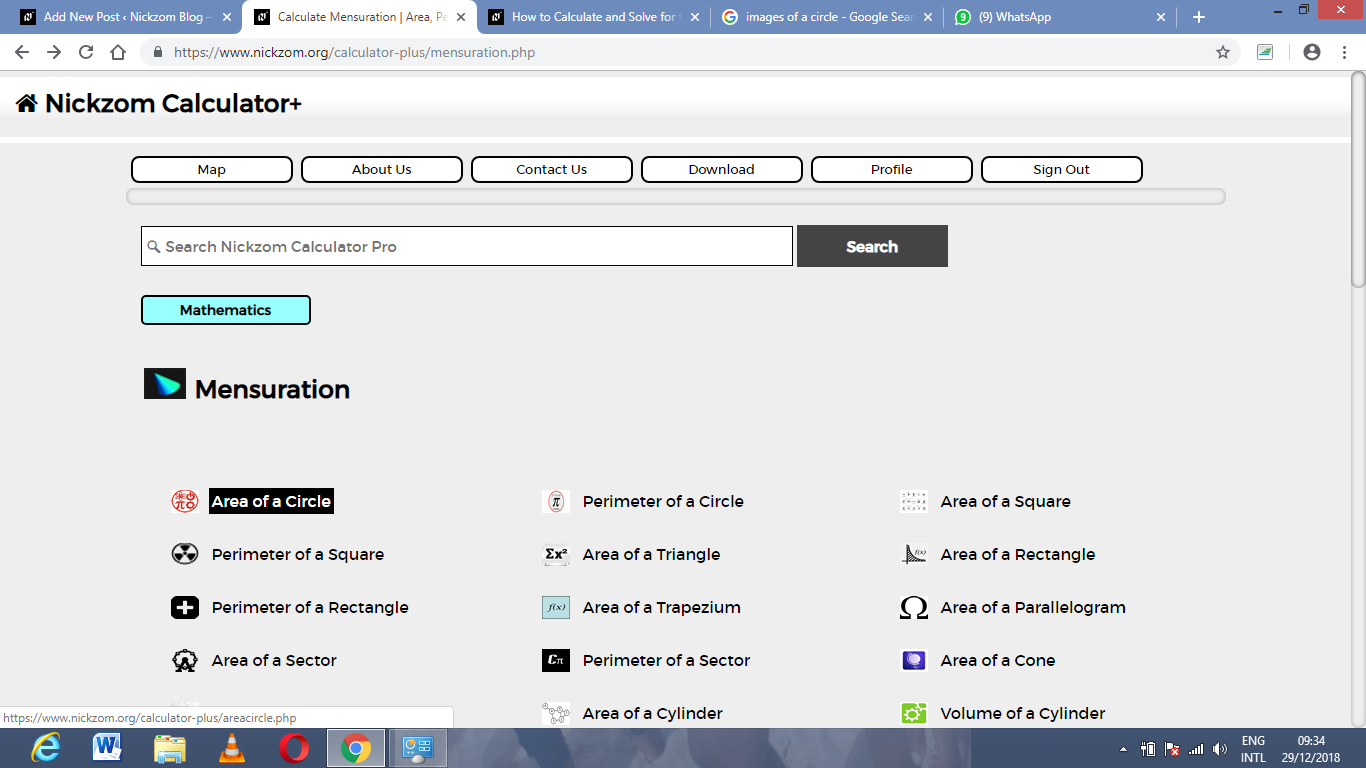The screenshot below displays the page or activity to enter your value, to get the answer for the area of a circle according to the respective parameter which is the radius of the circle (r).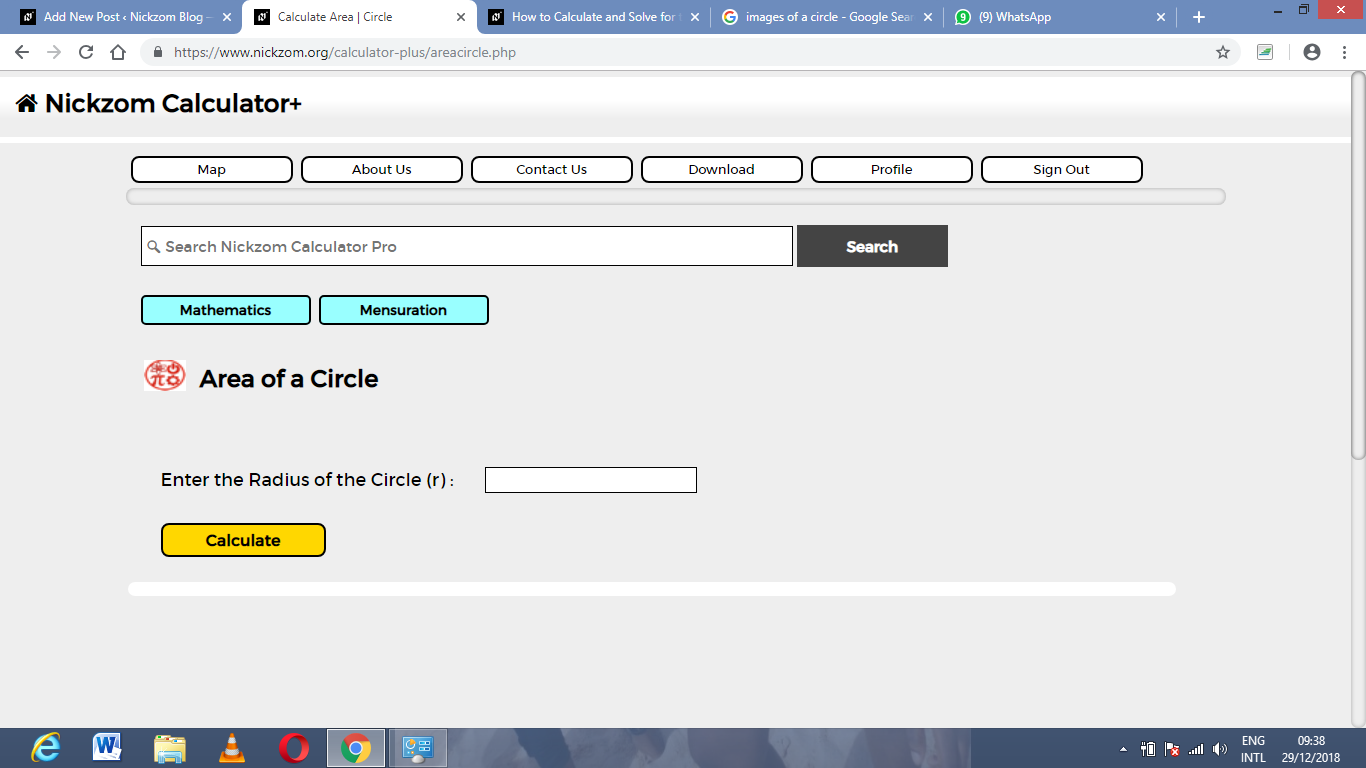Now, enter the value appropriately and accordingly for the parameter as required by the example above where the radius of the circle is 6.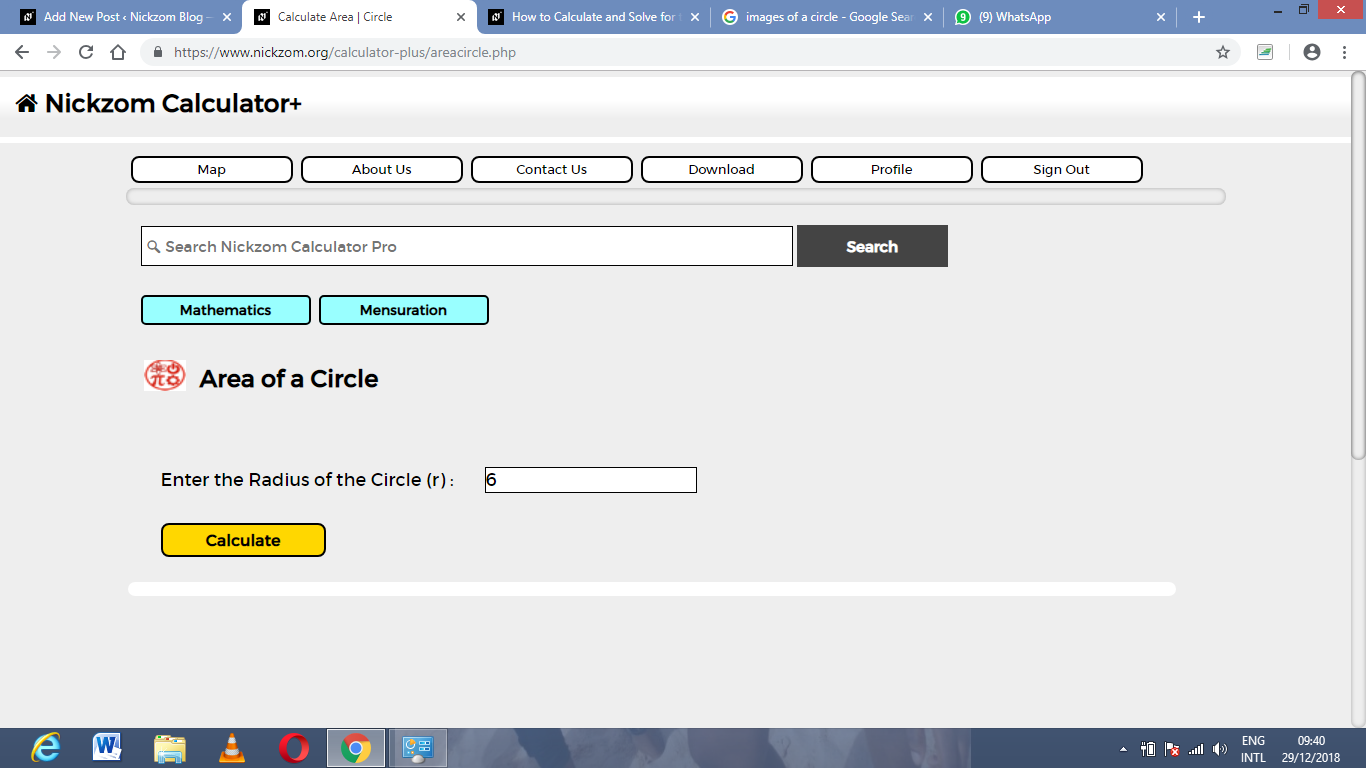Finally, click on Calculate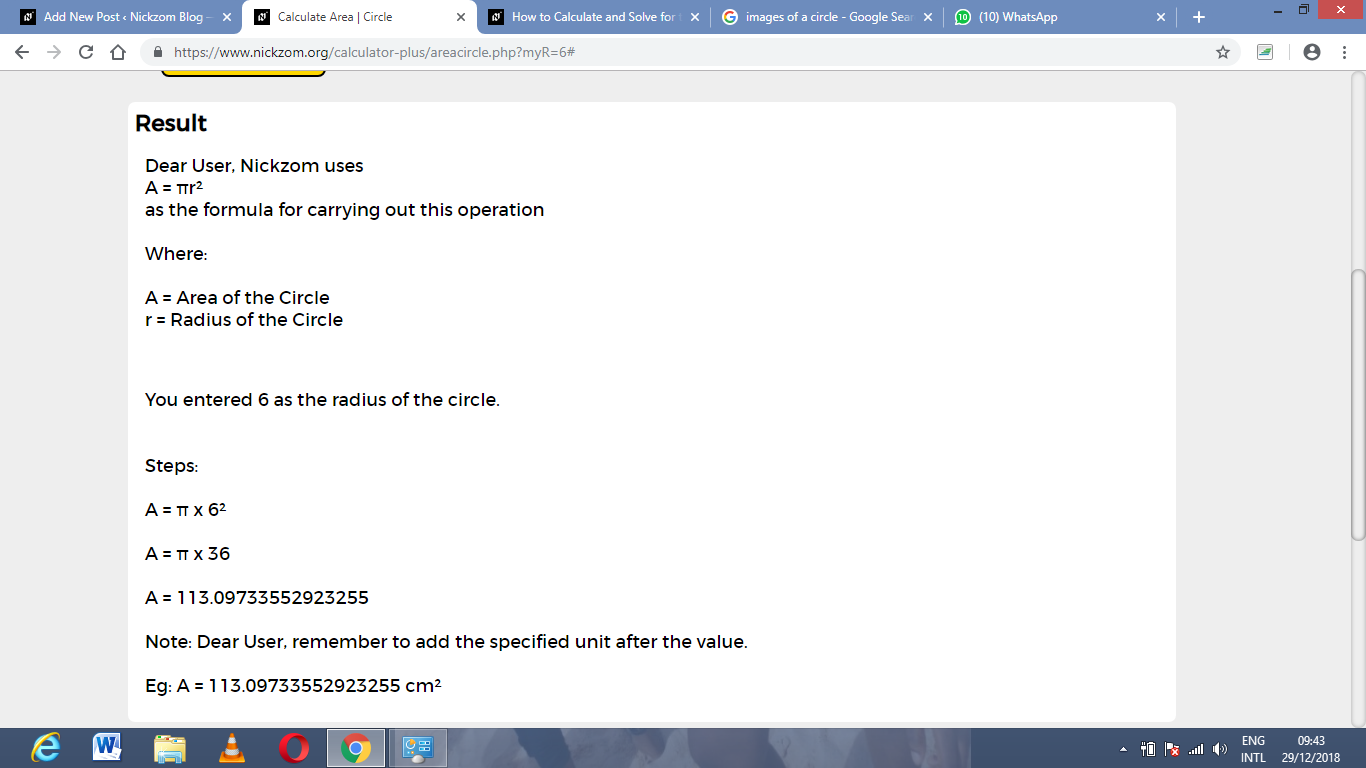As you can see from the screenshot above, Nickzom Calculator – The Calculator Encyclopedia solves for the area of a circle and presents the formula, workings and steps too.